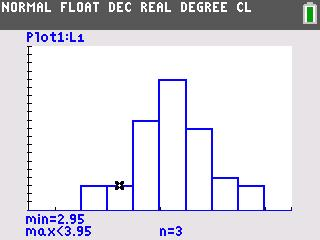### Modeling Probabilities

Students use simulations and graphs to explore what happens when the number of trials of a binomial experiment becomes a large number.
•TI-84 Plus CE
•TI-84 Plus C Silver Edition
• TI-84 Plus Silver Edition
• TI-84 Plus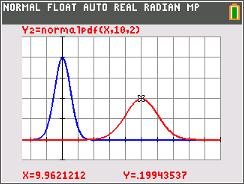### Average Orange

Students explore the area under the normal curve between various x-values and use a model to find what percent of the area lies within 1, 2, and 3 standard deviations of the mean. weights. Percentiles are defined and calculated.
•TI-84 Plus CE
•TI-84 Plus C Silver Edition
• TI-84 Plus Silver Edition
• TI-84 Plus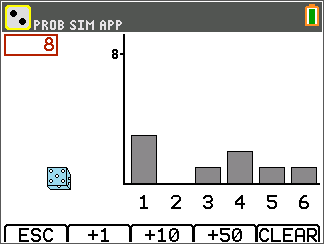### Binomial Probabilities

Students simulate rolling a die and keeping track of the numbers of successes and failures by using the randBin command.
•TI-84 Plus CE
•TI-84 Plus C Silver Edition
• TI-84 Plus Silver Edition
• TI-84 Plus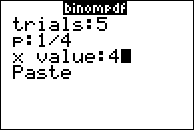### Binomial Probability in Baseball

In this activity, students will explore the link between Pascal's Triangle, the Binomial Theorem, and Binomial Probability Experiments.
•TI-84 Plus CE
•TI-84 Plus C Silver Edition
• TI-84 Plus Silver Edition
• TI-84 Plus### Permutations

Students are led through the development of the formula for finding n objects taken n at a time and n objects taken r at a time.
•TI-84 Plus CE
•TI-84 Plus C Silver Edition
• TI-84 Plus Silver Edition
• TI-84 Plus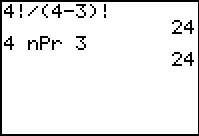### Permutations & Combinations

Students explore permutations and combinations by arranging letters when order does and does not make a difference.
•TI-84 Plus CE
•TI-84 Plus C Silver Edition
• TI-84 Plus Silver Edition
• TI-84 Plus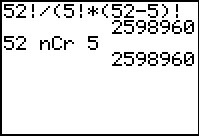••